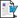# Physics 1 (2014/2015)Course code
4S00038
Name of lecturer
Pasquina Marzola
Coordinator
Pasquina Marzola
Number of ECTS credits allocated
6
FIS/01 - EXPERIMENTAL PHYSICS
Language of instruction
Italian
Location
VERONA
Period
II sem. dal Mar 2, 2015 al Jun 12, 2015.

#### Lesson timetable

II sem.
Day Time Type Place Note
Monday 10:30 AM - 12:30 PM lesson Lecture Hall A
Monday 2:30 PM - 3:30 PM lesson Lecture Hall A
Tuesday 8:30 AM - 10:30 AM lesson Lecture Hall Gino Tessari

#### Learning outcomes

This course is designed for students of the Master degree in Computer Science. The course will be focussed on the foundations of the experimental method, the classical mechanics of a particle and systems of particles and thermodynamics.
The educational objectives will be reached through lessons and exercises; lessons will be held during the second semester for a total of 6 credits. To facilitate the student in understanding and learning the laws and principles of mechanics and thermodynamics, during the lectures, frequent recall to phenomenology will be performed. The course is completed by exercises in order to prepare students for final exams .

#### Syllabus

1- Physics and measurement. Standards of lenght, mass and time. Dimensional analysis, Conversion of units. Vector and scalar quantities. Components of a vector and unit vectors. Properties of vectors. The scalar and vector products of two vectors.
2- Motion in one dimension. Position, velocity and speed. Particle under constant velocity. Acceleration. Motion diagrams. Particle under constant acceleration. Freely falling object. Motion in two dimensions. Position velocity and acceleration vectors. Two dimensional motion with constant acceleration. Projectile motion. Particle in uniform circular motion. Tangential and radial acceleration. Relative velocity and relative acceleration.
3- The Laws of Motion: The concept of force. Newton’s first law and inertial frames. Mass. Newton’s second law. The gravitational and electrostatic forces. The gravitational force and weight. Newton’s third law. Forces of friction. Newton’s second law for a particle under uniform circular motion. Non uniform circular motion. Motion in accelerated frames. Motion in the presence of resistive forces.
4- Energy and work. Work done by a constant force. Work done by a varying force. Kinetic energy and the work-kinetic energy theorem. Potential energy. Conservative and non conservative forces. Relationship between conservative forces and potential energy. Energy diagrams and equilibrium of a system. Conservation of energy for a non isolated system and for an isolated system. Situations involving kinetic friction. Changes in mechanical energy for non conservative forces. Power.
5- Linear momentum and collisions. Linear momentum and its conservation. Collisions in one dimension. Collisions in two dimensions. The center of mass. Systems of many particles. Rocket propulsion.
6- Rotation of a rigid object about a fixed axis. Angular position, velocity and acceleration. Rigid object under constant angular acceleration. Angular and translational quantities. Rotational kinetic energy. Moments of Inertia. Torque. Rigid object under a net torque. Energy consideration in rotational motion. Angular momentum. Angular momentum for a rigid object. Angular momentum for an isolated system.
7- Newton’s law of universal gravitation. Free-fall acceleration and the gravitational force. Kepler’s laws and the motion of planets. The gravitational field. Gravitational potential energy.
8- Fluid Mechanics. Pressure. Variation of pressure with depth. Pressure measurements. Archimede’s principle. Fluid dynamics. Bernoulli’s equation.
9- Oscillatory motion. Motion of an object attached to a spring. Particle in simple harmonic motion. Energy of the simple harmonic oscillator. Comparing simple harmonic motion with uniform circular motion. The pendulum. Damped and forced oscillations.
10- Thermodynamics. Temperature and the zeroth law of thermodynamics. Thermometers and the Celsius temperature scale. The constant-volume gas thermometer and the absolute temperature scale. Thermal expansion. Macroscopic description of an ideal gas. Heat and internal energy. Specific heat and calorimetry. Work and heat in thermodynamic processes. The first law of Thermodynamics. Heat engines and the second law of thermodynamics. Reversible and irreversible processes. The Carnot engine. Entropy.

#### Assessment methods and criteria

The final exam will be in written and will be focussed on solving typical problems of general physics (mechanics and thermodynamics) followed by an optional oral test. Necessary condition for passing the exam is to obtain a minimum mark of 18/30 in the written test. The oral test will be performed only for those students that have had in the written test a minimum mark of 26/30.
The dates of the exams are decided in harmony with the academic calendar approved by the Faculty.

Suggested book:
R.A. Serway, J.W. Jewett, Fisica per Scienze e Ingegneria vol I. Edizioni Edises.

#### Teaching aids

Documents
•diapo-cap15   (pdf, it, 1656 KB, 27/05/15)
•diapo-cap19   (pdf, it, 974 KB, 27/05/15)
•diapo-cap20   (pdf, it, 1168 KB, 27/05/15)
•diapo-cap22   (pdf, it, 1286 KB, 27/05/15)
•esame24giugno   (pdf, it, 167 KB, 26/06/15)
•esempi-esami   (pdf, it, 598 KB, 27/05/15)
•parziale Fisica I   (docx, it, 120 KB, 21/04/15)
•testo e svolgimento prova 8 giugno   (pdf, it, 169 KB, 08/06/15)